Contents

# Sampling and Analysis Details

## Gamma Measurement Details

All fish samples have been purchased from local fish markets in the Bay Area. Fish farmed or caught locally on the West Coast are given preference, in order to understand the local sea environment. However, we are also measuring samples of more inland animals like chicken
and pork, which people consume regularly.

### Sample Preparation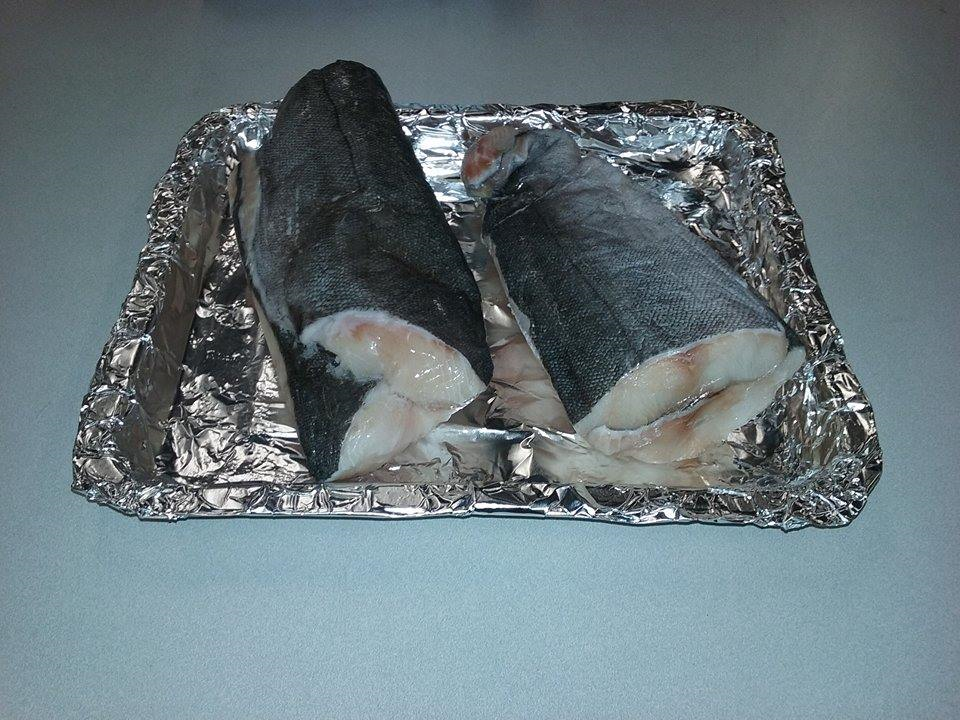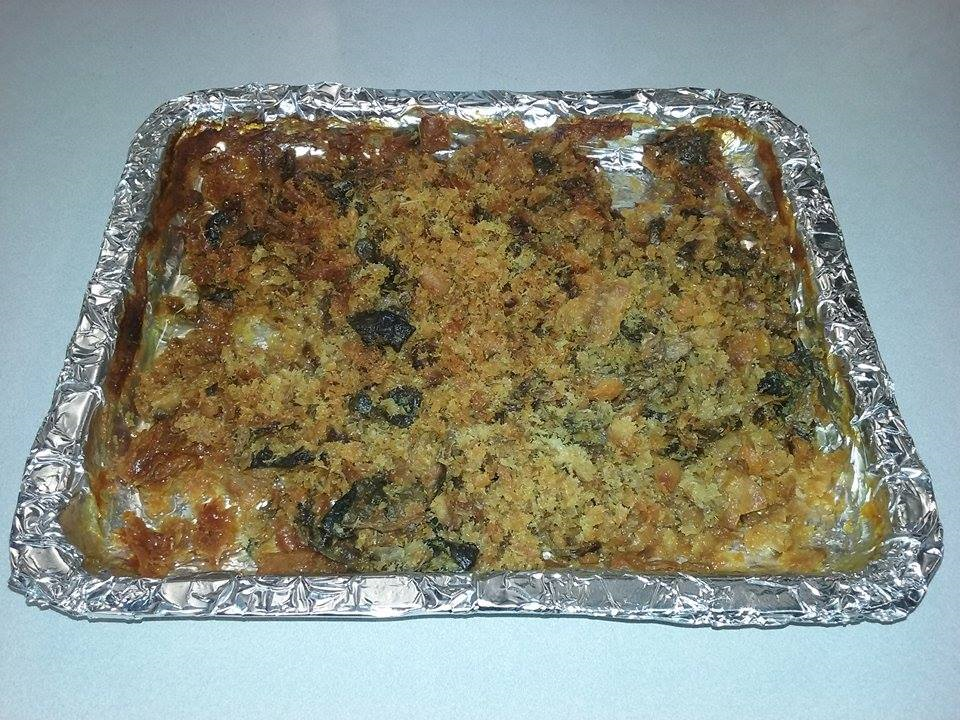Figure 1 (Left): One of our fish sample before drying process. Figure 2 (Right): The same fish sample after drying and cutting process.

Any liquid from water content or fats would attenuate the gamma radiation and weaken the radiation signal we're trying to detect. This moisture content is removed by baking the sample for a couple hours. Samples are continually cut with a clean knife to speed up the drying process and make it easier to fit inside a Marinelli beaker (see Figure 1-2). Once samples are dry, they are then placed in a Marinelli beaker and weighed. The wet weight is provided by the local fish market. The dry mass is collected after sample processing and is used in radioisotope calculations.Figure 3: Marinelli beaker with dried sample inside. The label indicates what type of food sample, the date it was measured, and its wet and dry mass.

### Data Collection

Samples are placed in front of a high purity Germanium (HPGe) detector and measured for a day. The day long measurement is for good statistics and to see slow-building features that wouldn't be noticeable in a shorter measurement.Figure 4: Sample placed into lead chamber. The front of the lead cave is open in order to place the sample. During measurements, this is closed up using additional lead bricks.

When the measurement is finished, an energy spectrum is collected, like the one in Figure 5. We search for peaks in the energy spectrum that correspond to radioisotopes of interest. Each radioisotope has its own set of gamma ray emissions that are listed in the table below.Figure 5: Characteristic Energy spectrum obtained from samples measured by the HPGe.

### Analysis Procedure

We search for peaks in the energy spectrum that correspond to radioisotopes of interest. Each radioisotope has its own set of gamma ray emissions that are listed in Table 1.

IsotopeEnergy (keV)Gamma Branching Ratio (%)
Cs-134604.7297.62
Cs-137661.6685.1
K-401460.8310.81
Bi-214609.3145.49
1120.2914.91
1764.4915.28
Tl-208583.1930.6
2614.5135.9

Simply finding the peaks and summing the total counts is not sufficient for calculations. This is due to Compton scattering that can occur inside the detector material. Gamma radiation can scatter inside the detector, which can contribute counts at energies below its full energy.
Since there are multiple gamma rays from various radioisotopes, a Compton background builds up in the spectra, with a noticeable 'plateau' coming from gammas associated with K-40.

This compton background must be subtracted from the total peak area in order to know the contribution purely from the radioisotope itself. This is done by looking at counts in regions around the peak and estimating the Compton background. Subtracting this from the total peak area gives us our net area contributed by the radioisotope gamma ray. An example of this procedure is illustrated in Figure 6.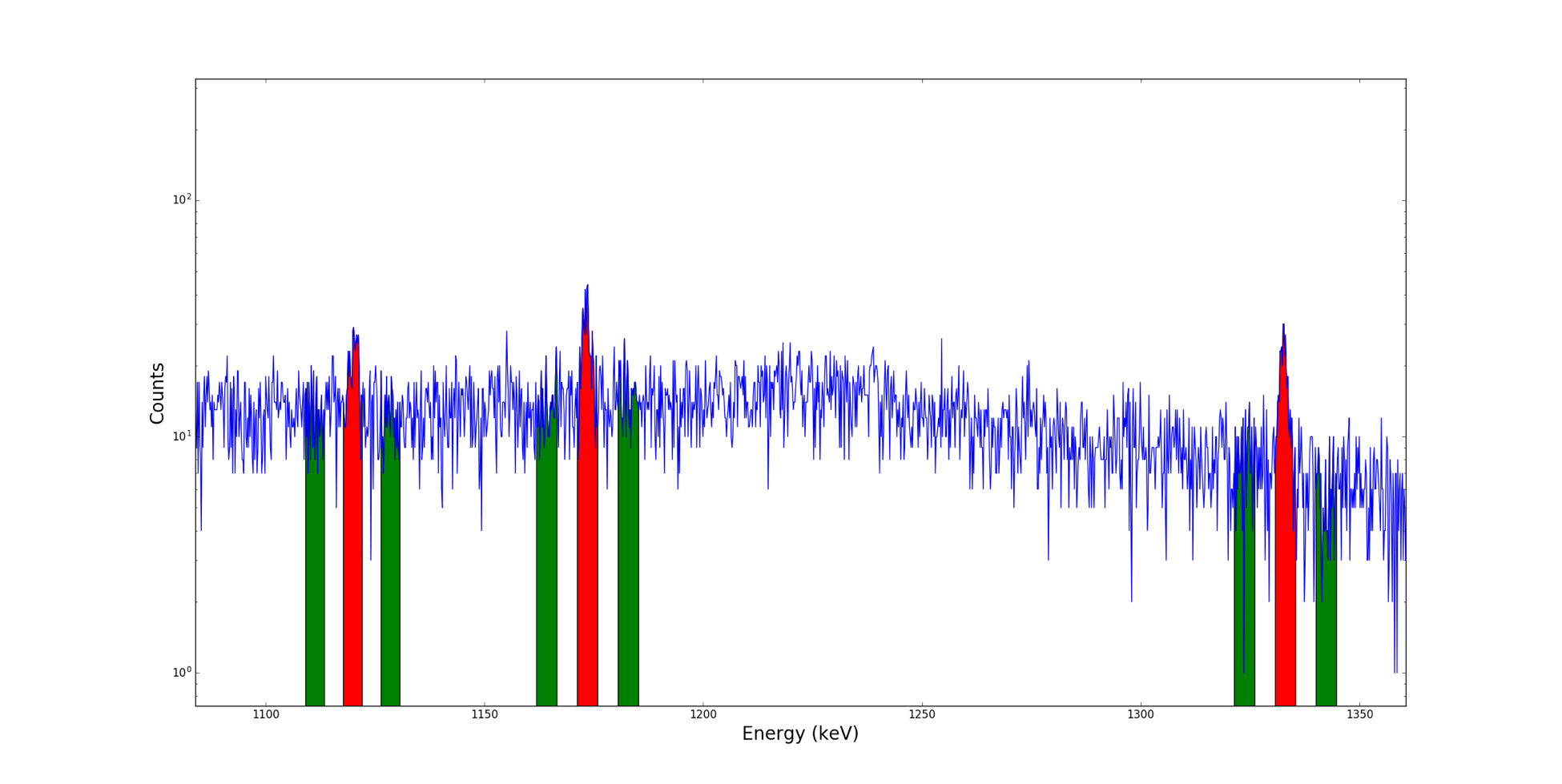Figure 6: Illustration of the side-band procedure for determining the Compton background under a peak of interest. The two side-band regions around each peak are indicated in green and the peaks of interest in red. The side-bands around each peak are averaged to estimate the background under the peak.

Subtraction of the Compton background alone is not sufficient to evaluate concentrations. We also need to account for background from the environment around the detector. Even though the detector is inside a lead chamber, small amounts of radiation can still get through. To account for this, we take a background spectrum by measuring an empty chamber. This measurement is normally taken for a longer period of time in order to measure more precisely the count-rates at all energies.Figure 7: Characteristic Energy spectrum from background for the HPGe under the same conditions as when sample measurements are taken.

### Activity Determination

Once we subtract the background, we then can calculate the count-rate from our sample by dividing the net area by the measurement time (specifically, the time the detector was active or “live”). The countrate enables us to calculate the activity of a certain radioisotope
using the following relation:

$$Activity = \frac{cps}{\epsilon * \beta}$$

Where $$cps$$ is the measured count-rate, $$\epsilon$$ is the detector efficiency at a specific energy, and $$\beta$$ is the branching ratio, i.e. the probability for a given radioisotope to produce a gamma ray at that energy. We know the efficiency of our detector at all energies
for samples in the marinelli beaker. The above relation allows us to evaluate the activity within a given food sample.

For the case of K-40, Bi-214, and Tl-208, these are naturally occurring isotopes that can be more accurately quantified with the use of a reference sample. The reference sample has known quantities of these three isotopes and a measured countrate associated with them. Comparing
the sample countrate to the reference countrate allows us to be much more accurate in calculating these concentrations.

## Neutron Activation Analysis Details

Most of the analysis details related to the collection of energy spectra, peak finding, background subtraction, and activity determination for isotope identification and measurement in neutron activation analyses are the same as for passive gamma measurements as described above. Here we will focus on those details that differ, including the types of samples collected and the details of their preparation, as well as some specifics of how relative concentrations of heavy metals of interest are determined.

### Global Sample Collection

In order to obtain data worldwide, the team acquired samples from a variety of regions: South America, Asia, Europe, and the North Pacific Ocean. The samples were purchased in the Summer of 2016 at two markets local to the UC Berkeley campus. Tokyo Fish Market and 99 Ranch import aquatic life from various countries and were therefore selected to collect the diverse collection of samples. The team chose international samples to explore the elemental composition trends across the globe, such as abnormally high concentrations of an elements in a certain regions and other anomalies.

### Preparation and Irradiation of Samples

Samples are prepared for neutron activation in advance of being sent out for irradiation. Since water attenuates neutrons and stands as a barrier for proper neutron activation, the samples are baked to a crisp texture to eliminate any moisture, in a manner similar to that described above for the gamma analysis. The crisp sample is then crushed to a powder to fit it inside a vial about 1 cm in diameter and 2 cm in height, an example of this is shown in Figure 8. Each sample is placed inside two vials and the two vials were placed inside a larger
vial to keep the two equivalent samples together.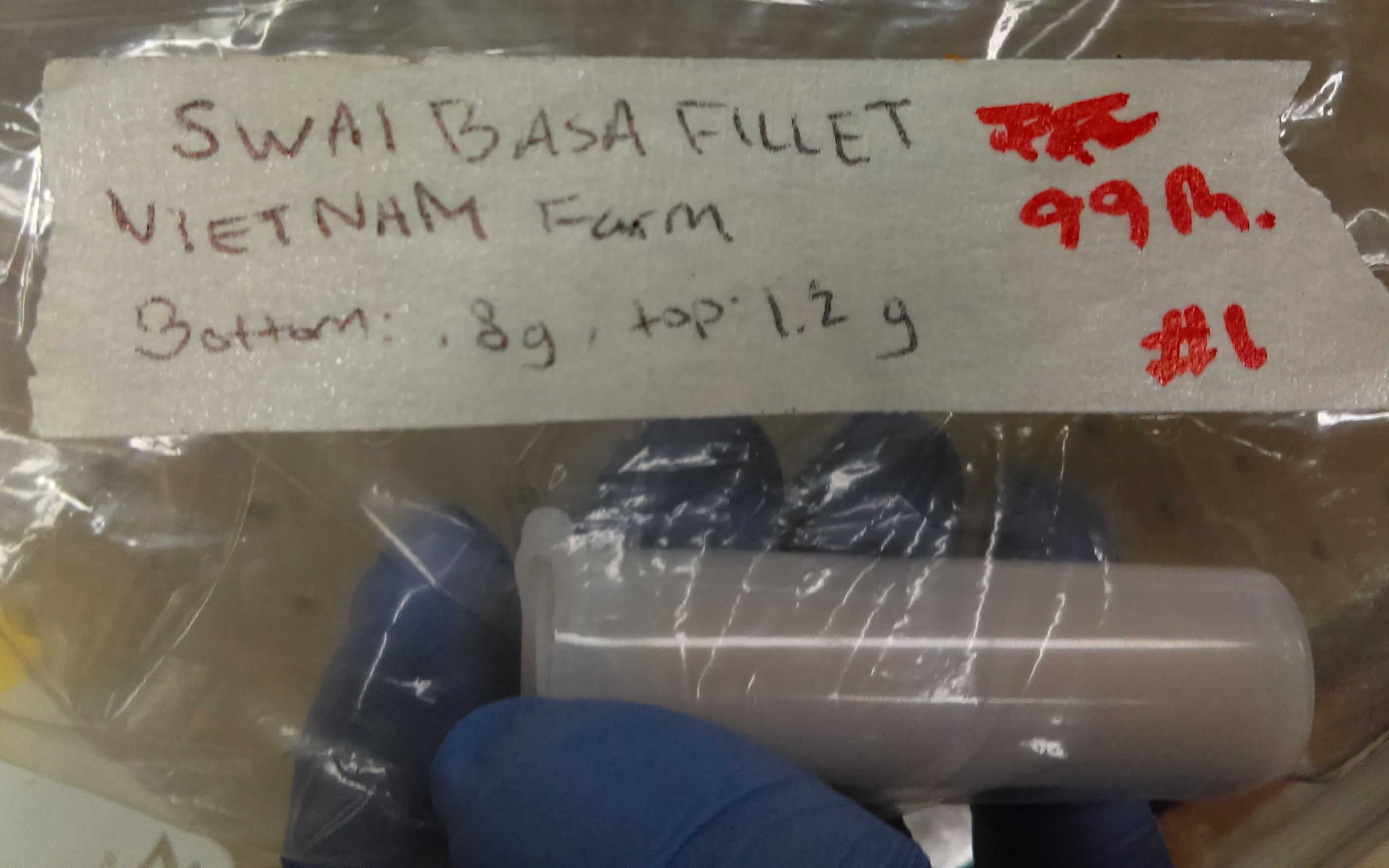Figure 8: Fish sample catalogued and ready for irradiation. Typically 1-4g of sample material are ground into a powder and placed in small vials in preparation for irradiation at McClellan Nuclear Research Center.

The prepared samples are taken to the McClellan Nuclear Research Center where the samples are then irradiated with thermal neutrons for ten minutes. After the samples are activated, they are mailed back to UC Berkeley after a couple days of “cooling down.” Once the samples are returned to the laboratory, they are measured using high purity germanium detectors. Figure 9 shows examples of how the sample vials are placed in front of the detector in a special sample mount that helps to ensure each sample is placed in the same location consistently. This helps
to minimize variations in the measurement geometry that would increase the systematics in our efficiency estimate.

To optimize the measurement sensitivity across short- and long-lived isotopes, samples are measured in batches for three different time intervals, 30 minutes, 3 hours, and 24 hours. First, each samples is measured for 30 minutes. After all of the 30 minute spectra are obtained
for a batch of samples, each sample is re-measured for 3 hours. After all of the 3-hour spectra are acquired, each sample undergoes a final 24 hour measurement. These spectra are combined to derive the full results for each sample. The three sets of spectra, with increasing integration
times, are collected to obtimize our sensitivy to activated isotopes across a range of half-lives and concentrations. Some of the heavy metals of interest have relatively short half-lives, meaning measurements taken very soon after the irradiation of the sample will have the greatest sensitivity to these isotopes and long integration times are not necessary. At the same time, longer integration times will increase our sensitivity to those heavy metals with relatively low concentrations in the measured sample.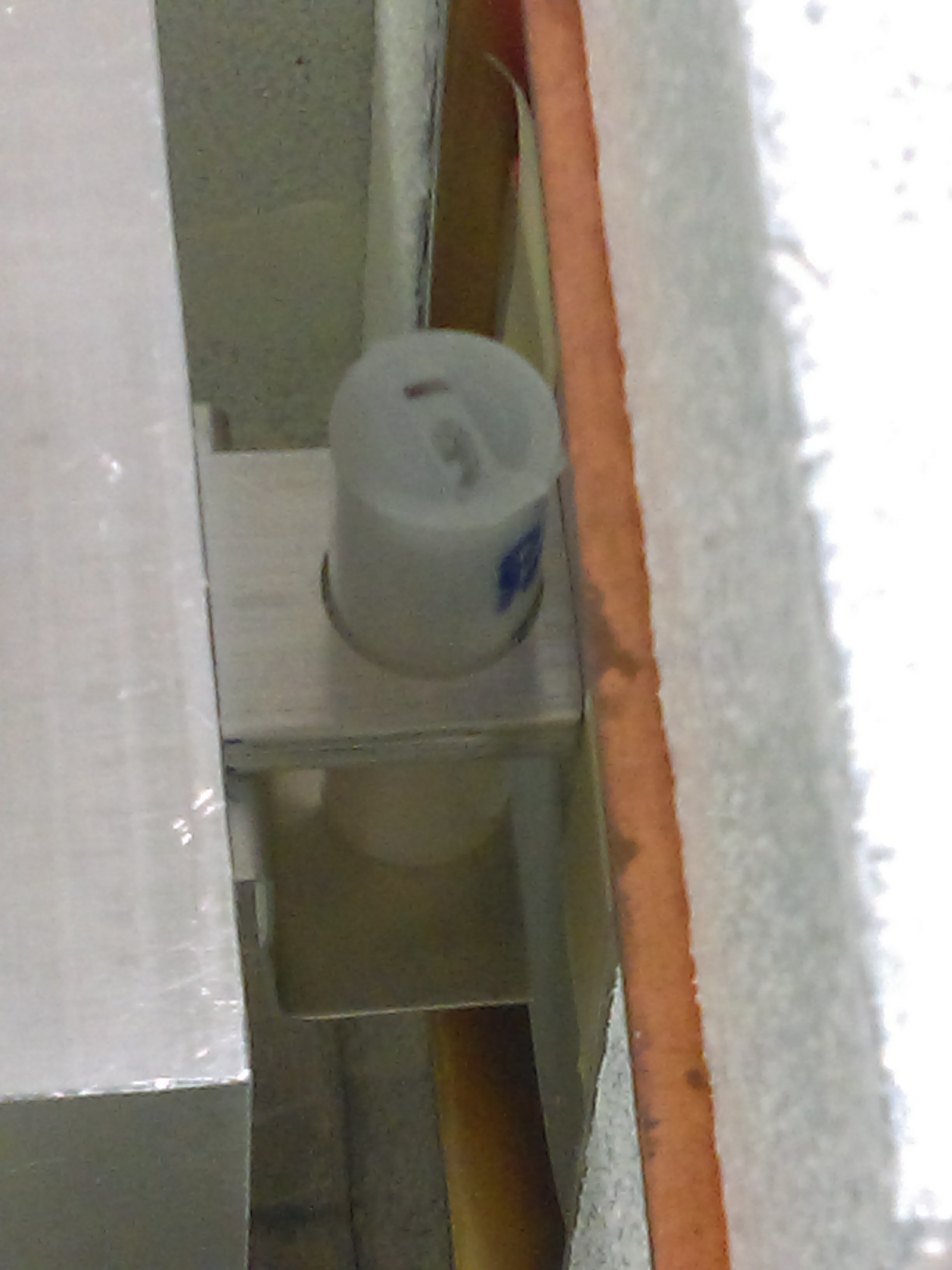Figure 9: Sample vials are placed in a specialized sample mount designed to hold the samples directly in front of the center of the detector face, always in the same position. The detector and samples are enclosed in a lead brick cave to minimize background radiation contributions to the measured spectra.

### Concentration Calculation

In order to calculate the efficiency needed to extract the decays per second for the samples, an efficiency curve was determined using six calibration sources: sodium, manganese, cobalt, cadmium, thorium, and europium. The efficiency curve helps find the decays per second from the detected counts per second since it allows the geometrical factor of the sample placement on the detector, as well as the sensitivity of the detector, to be taken into account. The band surrounding the efficiency curve indicates the systematic uncertainty propagated from the data
point on the curve.

The elemental weight for different isotopes is derived using the measured activity, A (as defined above) of the activated isotope, together with details of the irradiation process, to determine the total number of atoms, $$N_0$$, of the initial isotope and there-by the elemental
weight (elemental-weight = $$N_0/(N_A*A)$$):

$$N_0 = \frac{A}{\phi\sigma(1-exp(-\lambda t_{irr}))}$$

where $$\phi$$ is the irradiation flux, $$\sigma$$ is the activation cross-section, $$\lambda$$ is the activity coefficient of the activated isotope, and $$t_{irr}$$ is the length of the irradiation. To determine the relative concentration for that element in the sample, the elemental-weight is then scaled by the relative abundance for that isotope and the total sample weight.

The final uncertainty for each isotope in every sample includes the statistical uncertainty from the combined integrated counts, and a systematic uncertainty resulting from the uncertainty in the efficiency determination for the detector response and, when applicable, the uncertainty in the irradiation flux during activation. These uncertainties are shown separately as error bars and shaded bands respectively for each result. In comparisons of relative concentrations (ppm) in our samples with reference samples and environmental limits, these uncertainties were combined as a single total measurement uncertainty:

$$\sigma_{tot} = \sqrt{\sigma_{stat}^2 + \sigma_{sys}^2}$$

## Acknowledgements

The methodology for the Gamma measurements described here was developed primarily by Christopher Figueroa under the supervision of Dr. Brian Plimley. The NAA methodology was developed primarily by Jacqueline Garcia under the supervision of Dr. Plimley and Dr. Ali Hanks. This page is edited and maintained by Dr. Hanks.